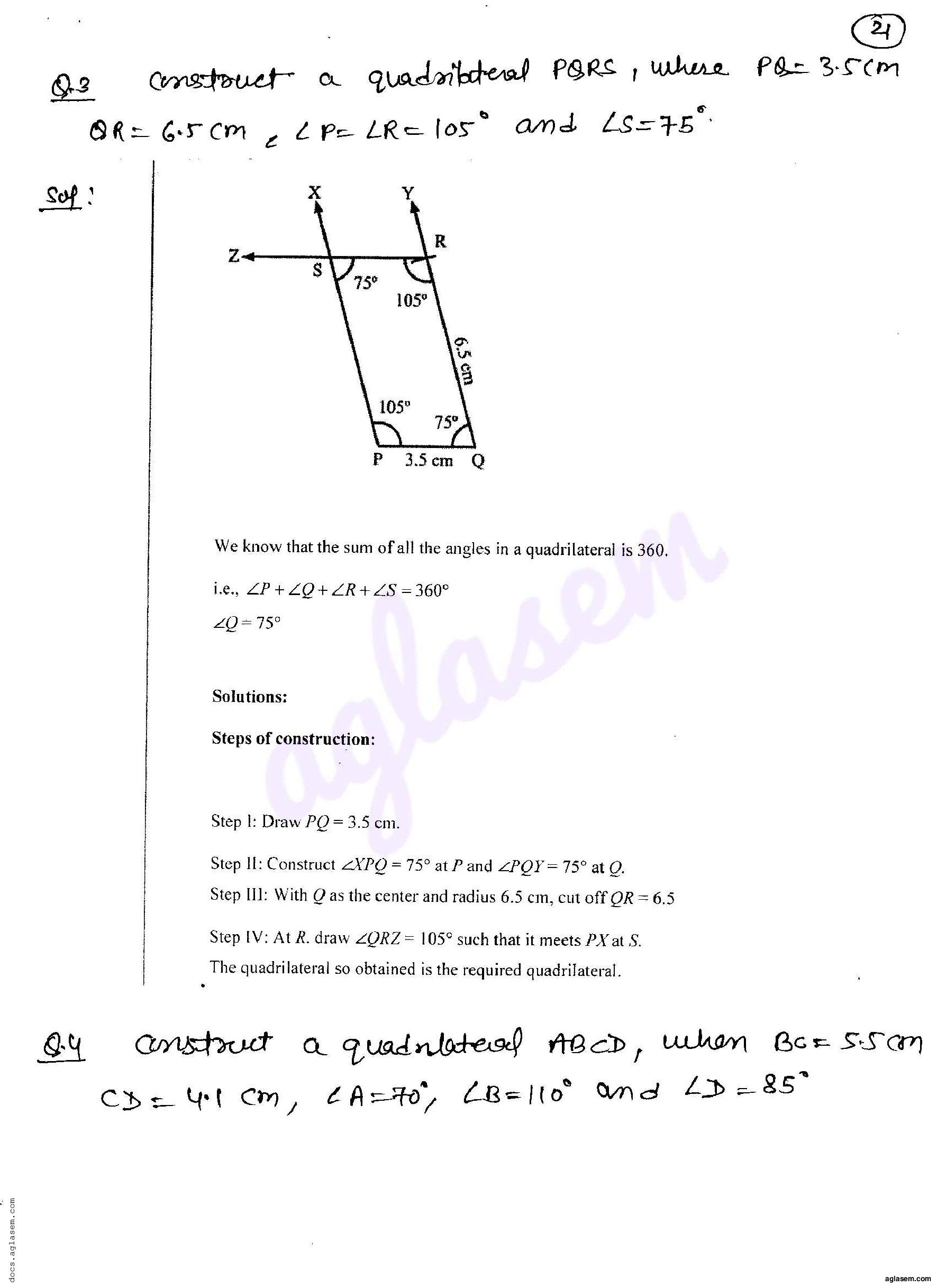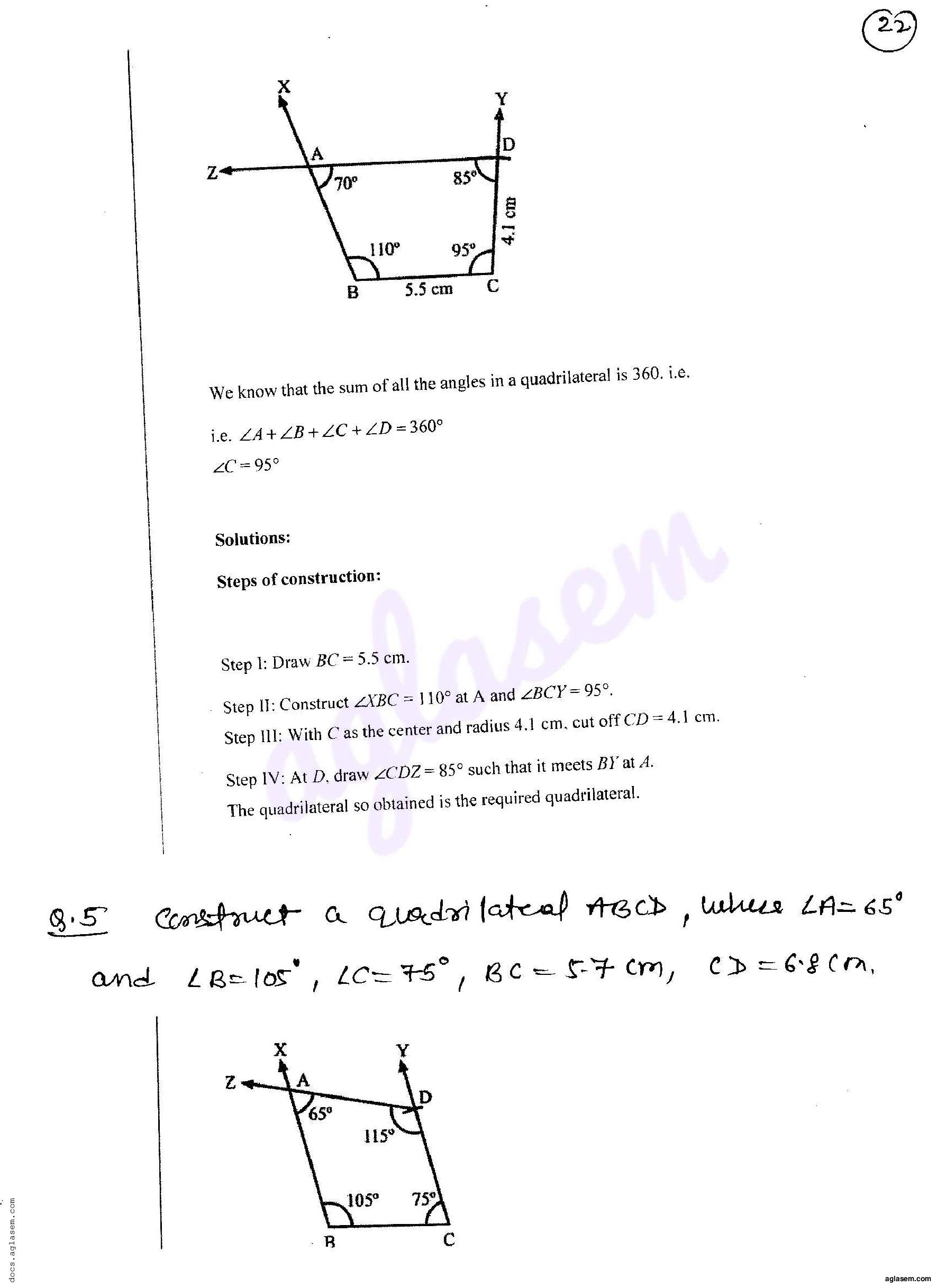# RD Sharma Solutions Class 8 Chapter 18 Practical Geometry (Constructions) Exercise 18.5

Here you can get free RD Sharma Solutions for Class 8 Maths Chapter 18 Practical Geometry (Constructions) Exercise 18.5. All RD Sharma Book Solutions are given here exercise wise for the chapter Practical Geometry (Constructions). RD Sharma Solutions are helpful in the preparation of several school level, graduate and undergraduate level competitive exams. Practicing questions from RD Sharma Mathematics Solutions for Class 8 Chapter 18 Practical Geometry (Constructions) is proven to enhance your math skills.

 Class: Class 8th Chapter: Chapter 18 Exercise: Exercise 18.5 Name: Practical Geometry (Constructions)

## RD Sharma Solutions Class 8 Chapter 18 Practical Geometry (Constructions) Exercise 18.5

RD Sharma Class 8 Solutions Chapter 18 for Practical Geometry (Constructions) Exercise 18.5 are given below.

RD Sharma Solutions Class 8 Chapter 18 Practical Geometry Constructions Exercise 18.5